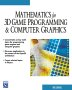# Maths - Matrix algebra - Inverse

### Division and Inverse matrix

We don't tend to use thenotation for division, since matrix multiplication is not commutative we need to be able to distinguish between [a][b]-1 and [b]-1[a].

One case where we can reverse the order is when the result is the identity matrix [I]

so for any matrix b then: [b][b]-1= [b]-1[b]= [I]

So instead of a divide operation we tend to multiply by the inverse, for instance if,

[m] = [a][b]

then,

[m][b]-1 = [a][b][b]-1

because [b][b]-1=[I] we can remove [b][b]-1 which gives:

[m][b]-1 = [a]

## Calculating inverse using determinants.

The inverse is the transpose of the matrix where each element is the determinant of its minor (with a sign calculation) divided by the determinant of the whole.

To calculate this we can follow these steps:

• Calculate the minor for every matrix element.
• Form the cofactor matrix from the minors with signs.
• Form the adjoint from cofactor matrix by transposing.
• Form the inverse by dividing the adjoint by the overall determinant.

For example a 3x3 matrices inverse is made up of the following determinants:

 m11 m12 m21 m22
 m02 m01 m22 m21
 m01 m02 m11 m12
 m12 m10 m22 m20
 m00 m02 m20 m22
 m02 m00 m12 m10
 m10 m11 m20 m21
 m01 m00 m21 m20
 m00 m01 m10 m11

divided by the of the matrix, where each of these terms is a determinant, expanding these out gives:

[m]-1 = 1/det[m] *
 m11*m22 - m12*m21 m02*m21 - m01*m22 m01*m12 - m02*m11 m12*m20 - m10*m22 m00*m22 - m02*m20 m02*m10 - m00*m12 m10*m21 - m11*m20 m01*m20 - m00*m21 m00*m11 - m01*m10

## Inverse of some common transforms

Here are some examples of the inverse of

### rotate

In order to invert a rotation we just rotate by the same amount in the opposite direction. This is equivalent to swapping the rows with columns and columns with rows (see orthogonal matrices).

So, for example, the inverse of this:

[m] = rotate 90 degrees about Z axis =
 0 1 0 -1 0 0 0 0 1

is this:

[m]-1 = rotate -90 degrees about Z axis =
 0 -1 0 1 0 0 0 0 1

Try it in the 'inverse calculator'.

### translate

The inverse of a translation by (tx,ty,tz) is a translation by (-tx,-ty,-tz) just move it back in the opposite direction, The translate transform is often represented by a 4x4 matrix together with the multiplication operator as described here.

[m] = translate matrix =
 1 0 0 tx 0 1 0 ty 0 0 1 tz 0 0 0 1

So the inverse is just:

[m]-1 = inverse translate matrix =
 1 0 0 -tx 0 1 0 -ty 0 0 1 -tz 0 0 0 1

Try it in the 'inverse calculator'.

### scale

If we scale by Sx, Sy and Sz in the direction of the x,y and z axes, we get,

[m] = scale about x,y and z axes =
 Sx 0 0 0 Sy 0 0 0 Sz

So the inverse of this transform is:

[m]-1 = inverse scale about x,y and z axes =
 1/Sx 0 0 0 1/Sy 0 0 0 1/Sz

Try it in the 'inverse calculator'.

 metadata block see also: Correspondence about this page Book Shop - Further reading. Where I can, I have put links to Amazon for books that are relevant to the subject, click on the appropriate country flag to get more details of the book or to buy it from them.Mathematics for 3D game Programming - Includes introduction to Vectors, Matrices, Transforms and Trigonometry. (But no euler angles or quaternions). Also includes ray tracing and some linear & rotational physics also collision detection (but not collision response). Other Math Books Specific to this page here:

This site may have errors. Don't use for critical systems.